# Q.In Ship Stability for Masters and Mates by Capt D R Derrett ,law of floatation chapter 3 page 27,sixth edition while explaining hydrometer principle ,there is an equation 2 formed in order to equate the volume of water displaced in density of water rho,which has been divided by 1000. Kindly please tell why the 1000 has been divided?

I suppose it is about the "Variable Immersion Hydrometer". Let me re-phrase it.

The section in the book is talking about how the marking are made (Calculated) on the Hydrometer.  Hydrometer is made to float in fresh water at Bottom marking (X) and then at Upper marking (Y) by adding the weight to its bulb.

Mx and My are the respective measured weights.  Now with weight of the Hydrometer being My, it is placed in water with unknown density (rho). We need to find this density which after calculation will be marked on the hydrometer.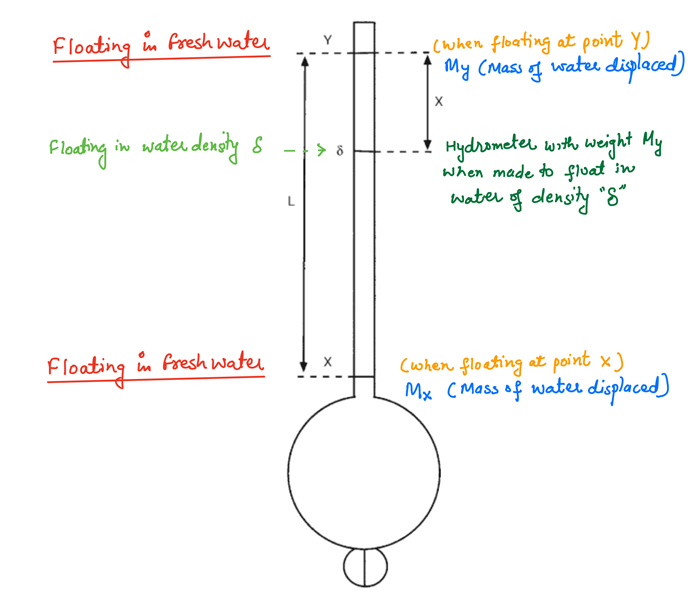This is done in few steps. First the area of the Stem between point X and Y is calculated. Which is simply is Volume divided by Length (L).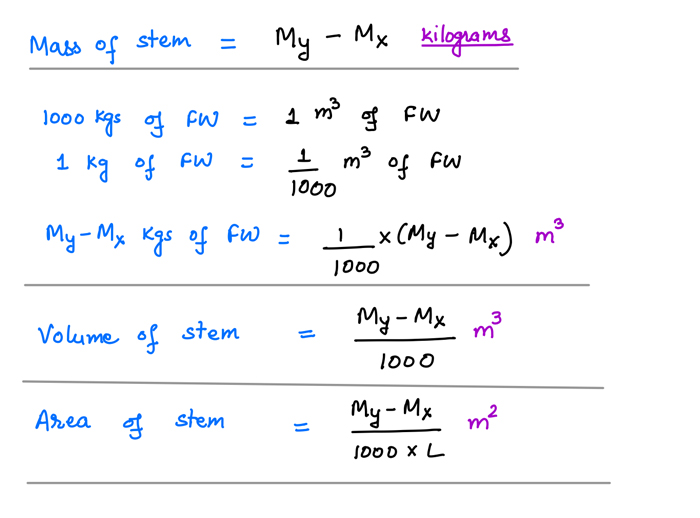Next is to get the Volume of water displaced in this density.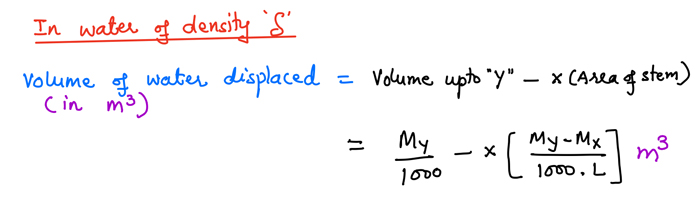Now here comes the part of your confusion.

Volume is also equal to the Weight divided by the density. But the important thing is to take care of the units. If we are measuring Volume in m3 and weight in Kg then density need to be Kg/m3. Or if the unit of the density is ton/m3 then the weight need to be in Tons which will give the volumes in m3 by this formula.

For this excercise, Weight "My" is in Kilograms (Kgs), if we are using unit of the density as Tons/m3 then we need to convert "My" to tons which we can do by dividing it by 1000.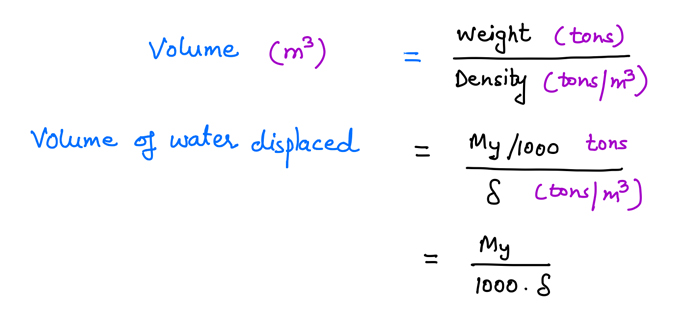That would have removed your confusion but I will complete the exercise for the sake of it.

Comparing two equations for Volume of water displaced at density (rho).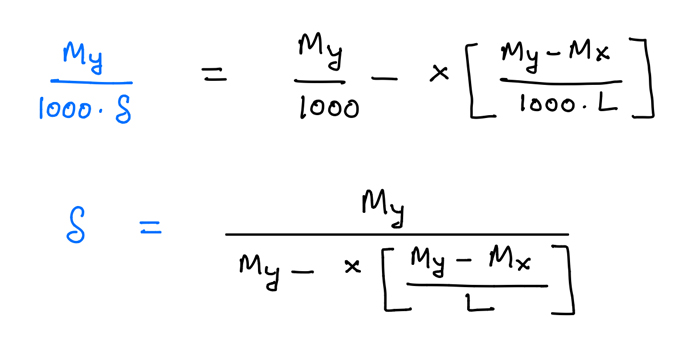In this equation the only unknown values are the density and length (L). So for example if we want to know the position of marking on hydrometer for a particular density (say 1.010) then we can get the value of L and thus the position where this need to be marked on the hydrometer.

The main point to take home is that when dealing with ship stability always consider the units on both side of the equation. If considered, the confusion will not arise.